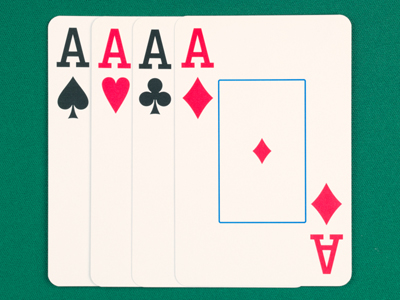There are four suits in a deck of cards.

# Verbal Reasoning - Complete the Sum 1

This Math quiz is called 'Verbal Reasoning - Complete the Sum 1' and it has been written by teachers to help you if you are studying the subject at elementary school. Playing educational quizzes is an enjoyable way to learn if you are in the 3rd, 4th or 5th grade - aged 8 to 11.

It costs only \$12.50 per month to play this quiz and over 3,500 others that help you with your school work. You can subscribe on the page at Join Us

In this quiz you have to find the number that will complete the sum correctly. The only operations that will be used are multiply, divide, add and subtract. Choose the correct answer from the four choices available.

Sometimes you have to work out the sum to find the last number, and sometimes you have to find a number in the middle of the sum. An example has been done for you (see below and read it carefully so you understand before playing the quiz).

Example:

26 + 7 = 18 + ?

13
14
15
16

This is because 26 + 7 = 33. 18 + ? = 33. This makes 15 the only possible answer.
1.
Find the number that will complete the sum below correctly.

? + 26 = 30 + 4.
8
9
10
11
This is because 30 + 4 = 34. To turn ? + 26 into 34 we need to add 8. Here's an interesting fact for you: a number is always divisible by 8 if its last three digits are also divisible by 8, for example 6,240
2.
Find the number that will complete the sum below correctly.

45 - ? = 13 x 3
7
6
5
4
This is because 45 - 6 = 39, which is the same as = 13 x 3. Some people consider 13 to be an unlucky number and Friday 13th is thought to be an unlucky date. Any month's 13th day will fall on a Friday if the month begins with a Sunday
3.
Find the number that will complete the sum below correctly.

? ÷ 9 = 15 - 7
76
64
58
72
This is because 15 - 7 = 8 and 72 ÷ 9 = 8
4.
Find the number that will complete the sum below correctly.

35 - ? = 4 x 4
16
17
18
19
This is because 4 x 4 = 16. This is equal to 35 - 19. When we multiply a number by itself, the product is known as the square of that number. So 16 is the square of 4. Another example is 25 which is the square of 5
5.
Find the number that will complete the sum below correctly.

14 ÷ 2 = 63 ÷ ?
7
5
9
6
This is because 14 ÷ 2 = 7 and 63 ÷ 9 is also equal to 7. Can you remember what a prime number is? Good. Well, 2 is the only prime number which is also an even number
6.
Find the number that will complete the sum below correctly.

18 - 5 = 78 ÷ ?
6
5
7
4
This is because 18 - 5 = 13. To get from 78 to 13 we need to divide it by 6
7.
Find the number that will complete the sum below correctly.

56 x 2 = 127 - ?
12
13
14
15
This is because 127 - 15 = 112 which is equal to 56 x 2
8.
Find the number that will complete the sum below correctly.

12 x 4 = 52 - ?
6
3
4
8
This is because 12 x 4 = 48 and 52 - 4 is also 48
9.
Find the number that will complete the sum below correctly.

19 x 4 = ? + 52
25
24
23
26
This is because 24 + 52 = 76. 19 x 4 also gives the answer of 76. Many things are arranged in fours. A deck of cards has four suits, there are four points of a compass and, if you're lucky, there are four leaves on a clover!
10.
Find the number that will complete the sum below correctly.

17 + ? = 49 - 13.
18
19
17
21
This is because 49 - 13 is 36. The answer must be 19 as 17 + 19 = 36. 19 is a prime number. A prime number is a number which is only divisible by itself and 1
Author:  Stephen O'Hara